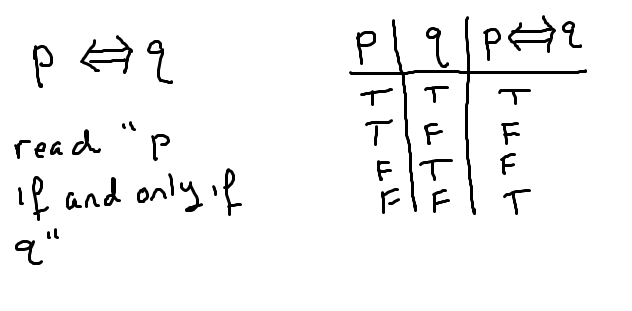# Truth tables – the conditional and the biconditional (“implies” and “iff”)

Just about every theorem in mathematics takes on the form “if, then” (the conditional) or “iff” (short for if and only if – the biconditional). Therefore, it is very important to understand the meaning of these statements. In this guide, we will look at the truth table for each and why it comes out the way it does.

As we analyze the truth tables, remember that the idea is to show the truth value for the statement, given every possible combination of truth values for p and q. Therefore the order of the rows doesn’t matter – its the rows themselves that must be correct. For each truth table below, we have two propositions: p and q. They can either both be true (first row), both be false (last row), or have one true and the other false (middle two rows). Writing this out is the first step of any truth table.

## The conditional – “p implies q” or “if p, then q”The conditional statement is saying that if p is true, then q will immediately follow and thus be true. So, the first row naturally follows this definition. Similarly, the second row follows this because is we say “p implies q”, and then p is true but q is false, then the statement “p implies q” must be false, as q didn’t immediately follow p.

The last two rows are the tough ones to think about. So let’s look at them individually.

• Row 3: p is false, q is true.
Think of the following statement. If it is sunny, I wear my sunglasses. If p is false, and q is true, then this is saying that it is not sunny, but I wore my sunglasses anyway. This certainly doesn’t invalidate my original statement as I might just like my sunglasses. So if p is false, but q is true, it is reasonable to think “p implies q” is still true.

• Row 4: p is false, q is false.
Using the example about sunglasses above, this would be equivalent to it not being sunny and me not wearing my sunglasses. Again, this wouldn’t invalidate my statement that “if it is sunny, I wear my sunglasses”. Therefore, if p is false and q is true, “p implies q” is still true.

Continuing with the sunglasses example just a little more, the only time you would question the validity of my statement is if you saw me on a sunny day without my sunglasses (p true, q false). Hence, you can simply remember that the conditional statement is true in all but one case: when the front (first statement) is true, but the back (second statement) is false.

## The biconditional – “p iff q” or “p if and only if q”If and only if statements, which math people like to shorthand with “iff”, are very powerful as they are essentially saying that p and q are interchangeable statements. When one is true, you automatically know the other is true as well. Also, when one is false, the other must also be false. This is reflected in the truth table. Whenever the two statements have the same truth value, the biconditional is true. Otherwise, it is false.

The biconditional uses a double arrow because it is really saying “p implies q” and also “q implies p”. Symbolically, it is equivalent to:

$$\left(p \Rightarrow q\right) \wedge \left(q \Rightarrow p\right)$$

This form can be useful when writing proof or when showing logical equivalencies.